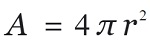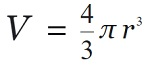# C program to calculate the surface area, volume of the Sphere

Here, we are going to learn how to calculate the surface area, volume of the Sphere using C program?
Submitted by Nidhi, on August 10, 2021

Problem Solution:

Read the radius of Sphere from the user, and then calculate the surface area and volume of the Sphere.

Surface area of the Sphere:

The formula to calculate the surface area of the Sphere is:Volume of the Sphere:

The formula to calculate the volume of the Sphere is:Program:

The source code to calculate the surface area, volume of the Sphere is given below. The given program is compiled and executed using GCC compile on UBUNTU 18.04 OS successfully.

```// C program to calculate the surface Area and
// volume of Sphere

#include <stdio.h>
#include <math.h>

int main()
{

float volume = 0;
float area = 0;

volume = (4.0 / 3) * (3.14) * pow(radius, 3);
area = 4 * (3.14) * pow(radius, 2);

printf("Volume of Sphere 	  : %f\n", volume);
printf("Surface area of Sphere: %f\n", area);

return 0;
}
```

Output:

```RUN 1:
Volume of Sphere          : 61.569649
Surface area of Sphere: 75.391403

RUN 2:
Volume of Sphere          : 4.186666
Surface area of Sphere: 12.560000

RUN 3:
Volume of Sphere          : 5572453.500000
Surface area of Sphere: 151976.000000
```

Explanation:

Here, we read the value of radius from the user. Then we calculated the surface area and volume of the Sphere using standard formulas.

What's New

Top Interview Coding Problems/Challenges!

Languages: » C » C++ » C++ STL » Java » Data Structure » C#.Net » Android » Kotlin » SQL
Web Technologies: » PHP » Python » JavaScript » CSS » Ajax » Node.js » Web programming/HTML
Solved programs: » C » C++ » DS » Java » C#
Aptitude que. & ans.: » C » C++ » Java » DBMS
Interview que. & ans.: » C » Embedded C » Java » SEO » HR
CS Subjects: » CS Basics » O.S. » Networks » DBMS » Embedded Systems » Cloud Computing
» Machine learning » CS Organizations » Linux » DOS
More: » Articles » Puzzles » News/Updates